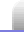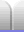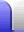# Physics Equations

Much of high school physics involves plugging the right numbers into the right equations. This page lists many of the equations used for Cambridge International Exams and NZ's own screwy system of NCEA. Use the email address at the bottom of the page for errors and omissions.

### Classical Physics

Value Equation Equation Explained Units
Density ρ = m/V density = mass / volume kg · m-3
Force F = ma
F = m∂v / ∂t
F = ∂(mv) / ∂t

F = ∂p / ∂t
force = mass * acceleration
...
force = change in momentum / change in time
N = kg · m·s-2

N = N·s · s-1
Impulse ∂p = F∂t = mv - mu impluse (change in momentum) = force * change in time = final momentum - initial momentum N·s = N · s
= kg · m·s-1
Momentum p = mv momentum = mass * velocity kg·m·s-1 = kg · m·s-1
Conservation of momentum m1u1 + m2u2 = m1v1 + m2v2 initial momentum (u is initial velocities) = final momentum (v is final velocities) kg · m·s-1 = kg · m·s-1
Equations of motion s = ½(u + v)t
s = ut + ½at²
s = vt – ½at²
v = u + at
v² = u² + 2as
• displacement = average velocity * time
• displacement = initial velocity * time + half acceleration * time squared
• displacement = final velocity * time – half acceleration * time squared
• final velocity = initial velocity + acceleration * time
• final velocity squared = initial velocity squared + twice acceleration * displacement

Equations of motion – differentials and integrals

v = ds / dt
a = dv / dt

s = ∫ v dt
v = ∫ a dt

velocity = change in displacement / change in time
acceleration = change in velocity / change in time

displacement = integral of velocity wrt time
velocity = integral of acceleration wrt time

There are also higher order quantities: change in acceleration per time is jerk; then jounce or snap; crackle; and pop.

Power P = W / t
P = Fd / t
P = Fv
power = work / time
power = force * distance / time
power = force * velocity
W = J · s-1
W = N · m · s-1
W = N · m·s-1
Pressure p = F / A pressure = force / area Pa = N · m-2
Pressure at depth (hydrostatic pressure) p = ρgh pressure = density * gravitational acceleration * depth Pa = kg·m-3 · m·s-2 · m
Work W = Fd work (energy) = force * distance (in the direction opposite the force) J = N · m
J = Pa · m3
Work done by a gas ΔW = pΔV
work = pressure * volume
This can be derived from the above work equation by multiplying it by area/area.
J = N · m
J = Pa · m3
Young Modulus E = σ / ∈
E = (F/A) / (x/L)
E = FL / Ax
Young modulus = stress / strain
= force per area / extension per length
= (force * length) / (area * extension)
Pa = Pa · [none]
= N · m-2 · m-1 · m
= N · m · m-2 · m-1

### Rotation, Circular Motion & Simple Harmonic Motion

Value Equation Notes Units
Angle θ = 2πt / T
= ωt
= s / r
swept angle = 2π * time / period
= angular velocity * time
= m · m-1
Angular frequency ω = dθ / dt
= 2π / T
= 2πf
= v / r
angular velocity = change in angle over change in time = 2π / period = 2π * frequency = linear velocity / radius
This is the differential of angle w.r.t. time.
Also called angular speed, and angular velocity (although that one is a little different, being a pseudovector).
= rad per turn · Hz
= m·s-1 · m-1
Angular velocity of a spring in SHM ω = √ (k / m) angular velocity = square root of (spring constant / mass)
Angular acceleration α = dω / dt angular acceleration = charge in angular velocity over change in time
This is the differential of angular velocity w.r.t. time.
Angular momentum L = Iω angular momentum = rotational inertia * angular frequency kg·m2·s-1 = kg·m2 · rad·s-1
Angular momentum of thin shell cylinder L = mr2ω angular momentum = mass * radius squared * angular frequency kg·m2·s-1 = kg · m2 · rad·s-1
Centripetal force F = mv2 / r Centripetal force (centrifugal force) = mass * velocity squared / radius
This is simply F = ma with the formula for linear acceleration from below substituted in.
N = kg · (m·s-1)2 · m-1
Energy E = τθ energy = torque * angle
Hence torque can be given in joules per radian.
Dividing both sides by time gives power (see below).
Frequency f = 1 / T frequency = 1 / period Hz = s-1
Hooke's Law
(force on a spring)
F = -kX restoring force (hence the negative sign) is proportional to the displacement from equilibrium
Can also be stated without the negative sign, as the force required to produce the displacement. Each spring has its own value of k, the spring constant.
N = N·m-1 · m
Linear velocity v = 2πr / T
= rω
linear velocity = 2π * radius / period = radius * angular velocity
Also called tangential velocity.
Linear acceleration a = v2 / r linear acceleration = velocity squared / radius m·s-2 = (m·s-1)2 · m-1
Moments in equilibrium F1d1 + F2d2 = F3d3 + F4d4 sum of anticlockwise moments = sum of clockwise moments N · m = N · m
Pendulm T = 2π√(L / g) period = 2π * square root of (pendulum length / gravitational acceleration)
For very small swing angles.
s = [const.] * √(m / m·s-2)
Period T = 1 / f period = 1 / frequency s-1 = Hz
Power P = τω
E / t = τθ / t
power = torque * angular velocity
This is the scalar product of two vectors. Throw in 2π / 60 if using rpm, such as for a car engine.
Rotational inertia I = L / ω rotational inertia = angular momentum / angular frequency
Rotational inertia is also called moment of inertia.
kg · m2 = kg·m2·s-1 / rad·s-1
Rotational inertia of thin shell cylinder or pendulum I = mr2 rotational inertia = mass * radius (from pivot for pendulum) squared kg·m2 = kg · m2
... of solid cylinder I = ½mr2 rotational inertia = half * mass * radius squared kg·m2 = [const.] · kg · m2
... of thin shell sphere I = 2/3 mr2 rotational inertia = two thirds * mass * radius squared kg·m2 = [const.] · kg · m2
... of solid sphere I = 2/5 mr2 rotational inertia = two thirds * mass * radius squared kg·m2 = [const.] · kg · m2
... of long rod pivoted at centre I = 1/12 mL2 rotational inertia = one twelfth * mass * radius squared kg·m2 = [const.] · kg · m2
... of long rod pivoted at one end I = 1/3 mL2 rotational inertia = one third * mass * radius squared kg·m2 = [const.] · kg · m2
Rotational kinetic energy Ek = ½Iω2
kinetic energy = half * angular momentum * angular frequency squared J = [const.] · kg · (m·s-1)2
SHM displacement x = x0 sin ωt displacement = amplitude * sine( angular frequency * time )
Sine can be replaced with cosine (depending on start position of SHM).
m = m · [ratio]
SHM velocity v = ωx0 cos ωt
= ±ω √( x02 - x2 )
velocity = angular frequency * amplitude * cosine( angular frequency * time )
Cosine can be replaced with sine (depending on start position of SHM).
m·s-1 = rad·s-1 · m · [ratio]
SHM acceleration a = –ω2x
= –ω2x0 sin ωt
= –4π2f2x
acceleration = negative angular velocity squared * dispacement
The negative is because the acceleration is always in a direction opposite to the displacement from equilibrium.
Sine can be replaced with cosine (depending on start position of SHM).
This is closely related to Hooke's Law.
Torque τ = Fd torque = force * pependicular distance N·m = N · m
Torque τ = Iα torque = rotational inertia * angular acceleration
Assumes the body is not changing size, which would change the rotational inertia (see above).

### Electricity

In a circuit, emf (symbol V or E or ε) is the voltage from sources, while pd (symbol V) is the voltage dropped across resistances.

The electron volt is a unit of energy: electron volt = elementary charge * volts. 1 eV = 1 e * V (where e = 1.6 x 10-19 C).

Value Equation Notes Units
Current I = Q / t current = charge / time A = C · s-1
Current in a conductor I = nAve
• n is the number of available charge carriers (electrons) per cubic metre. This is a constant for a particular material (eg, copper).
• A is the cross-sectional area of the conductor (wire).
• v is the charge carrier (electron) drift velocity.
• e (also written as q) is the charge of each charge carrier (typically electrons).
A = m-3 · m2 · m·s-1 · C
Kirchhoff's First Law IT = I1 + I2 In a circuit, the total current flowing into a junction is equal to the total current flowing out of the junction. A
Kirchhoff's Second Law ∑emf = ∑pd In a circuit, around any loop the emf sum equals the pd sum. Alternatively, around any loop the sum of all emfs and pds equals zero. V
Ohm's Law V = IR voltage = current times resistance V = A · Ω
Power P = IV power = current * voltage W = A · V
Power P = I2R
P = V2 / R
power = current squared * resistance
power = voltage squared / resistance
These come from substituting Ohm's Law into P = IV.
W = A2 · Ω
W = V2 · Ω-1
Resistivity ρ = RA / L
R = ρL / A
resistivity = resistance * area / length
resistance = resistivity * length / area
Ω·m = Ω · m2 · m-1
Ω = Ω·m · m · m-2
Resistors in parallel 1/RT = 1/R1 + 1/R2
RT = ( 1/R1 + 1/R2 )-1
reciprocal of total resistance = sum of reciprocals of individual parallel resistances Ω-1

Ω
Resistors in series RT = R1 + R2 total resistance = sum of individual series resistances Ω
Resonant angular frequency ω = √(1 / LC) resonant angular frequency = square root of reciprocal of product of inductance and capacitance
Used for tuned (resonant) circuits.

Voltage V = Ep / Q voltage (potential difference) = potential energy per charge V = J · C-1

### Capacitors & Electric Fields

Value Equation Notes Units
Capacitance C = Q / V
= εA / d

Q = CV
V = Q / C
capacitance = charge / voltage
= permittivity * plate area / distance between plates
Where d is much smaller than the dimensions of the area of the plates. See permittivity below.
charge = capacitance * voltage
voltage = charge / capacitance
F = C·V-1
= F·m-1 · [none] · m-3 · m-1

C = F·V
V = C·F-1
Capacitors in parallel CT = C1 + C2 total capacitance = sum of individual parallel capacitances (because the plate area is added) Ω
Capacitors in series 1/CT = 1/C1 + 1/C2 reciprocal of total capacitance = sum of reciprocals of individual series capacitance Ω
Electric field between parallel plates E = V / d = F / Q electric field strength = voltage per distance = force per charge V·m-1 = N·C-1
Permittivity ε = ε0εr permittivity = permittivity of free space * relative permittivity
The relative permittivity is also called the dielectric constant, and is a dimensionless ratio, different for each dielectric material.
F·m-1= F·m-1 · [none]
Potential energy in uniform electric field
(ie, between parallel plates)
Ep = Fd = QV potential energy of a charge = voltage * charge
This comes from multiplying out the previous equation, or by rearranging the definition of the volt.
J = N·m = C·V
Electric potential energy stored in a capacitor Ep = ½QV
Ep = ½CV2
Ep = ½Q2 / C
potential energy = half * charge * voltage
= half * capacitance * voltage squared
= half * charge squared / capacitance
J = C·V
J = F·V2
J = C2·F-1

### Magnetism

Value Equation Notes Units
Flux linkage in solenoid Φ = BAN magnetic flux linkage = magnetic flux density * area * number of turns in solenoid Wb = T · m2 · [none]
Magnetic field,
flux density
B = φ / A
φ = BA
magnetic flux density = magnetic flux per area
magnetic flux = magnetic flux density * area
T = Wb · m-2
Wb = T · m2
Force on a conductor F = BILsinθ force = magnetic flux density * current * length of conductor (in field) * sine of angle N = T · A · m · [none]
Force on a moving charge F = Bqvsinθ
F = Bevsinθ
(for electron)
force = magnetic field * charge * velocity of particle * sine of angle
N = T · C · m·s-1 · [none]

### Nuclear physics

Value Equation Notes Units
Energy-mass equivalence E = mc2 energy = mass * speed of light squared
J = kg · (m·s-1)2

### Optics & Waves

Speed of light in air or vacuum = c = 3 x 108 m/s. (This is the two-way speed of light because it is impossible to measure the one-way speed of light.)

Permittivity of free space = ε0 = 8.85 x 10-12 F·m- 1 (or C2·N-1·m-2 or C·V-1·m-1).

Permeability of free space = µ0 = 4π x 10-7 H ·m-1 = 12.6 x 10-7 H·m-1 (or N·A-2).

Value Equation Notes Units
Doppler change f = f0(v±vr)/(v±vs)

λ = λ0(v±vs)/(v±vr)
f; λ = observed frequency; wavelength
f0; λ0 = original frequency; wavelength
v = velocity of waves
vs = source velocity
The observed frequency is increased if the source and receiver are moving toward each other, reduced if they are moving apart.
Note the reversal of vr and vs for wavelength.
Hz = Hz · (m·s-1)

m = m · (m·s-1)
Period, frequency T = 1 / f
f = 1 / T
period (the time for one full wave) is the reciprocal of the frequency s = 1 / Hz
Hz = 1 / s
Speed of light c = 1 / √(ε0µ0) the speed of light is the geometric mean of the permittivity of free space and the permeability of free space (aka the electric constant and the magnetic constant respectively) m·s-1 = 1 / √ (F·m-1 · H·m-1)
Speed, frequency, wavelength c = fλ
v = fλ
speed of light = frequency * wavelength (em radiation in air)
speed = frequency * wavelength (other situations such as sound)
m·s-1 = Hz · m
Refractive index n = c / v refractive index = speed of light / speed of light in medium; hence minimum refractive index is 1. [none] = m·s-1 / m·s-1
Snell's Law n1sinθ1 = n2sinθ2 refractive index 1 * sine of angle 1 = refractive index 2 * sine of angle 2 [none]
Diffraction grating equation nλ = dsinθ
nλ = sinθ / N
d = line separation in diffraction grating
N = number of lines per metre in diffraction grating

Twin slit equation nλ = dx / L d = slit separation; x = fringe separation; L = distance to screen
This is a version of the above equation. For very small angles sinθ ≈ tanθ, and tanθ = x / L.Home Astronomy Chemistry Electronics & Computers Mathematics Physics Field Trips Turn on javascript for email link